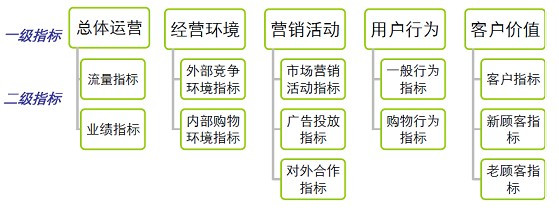# 熵

Entropy来源于希腊语，原意：内向，即：一个系统不受外部干扰时往内部稳定状态发展的特性。熵是热力学的一个物理概念，定义的其实是一个热力学的系统变化的趋势

$\Delta S = \frac{Q}{T} = \frac{热量}{温度} \tag{1-1}$

• 广义的定义：熵是描述一个系统的无序程度的变量；同样的表述还有，熵是系统混乱度的度量，一切自发的不可逆过程都是从有序到无序的变化过程，向熵增的方向进行。熵越大说明系统越混乱，携带的信息越少，熵越小说明系统越有序，携带的信息越多。

• 信息论中，熵是接受的每条消息中包含的信息的平均值。又被称为信息熵、信源熵、平均自信息量。

1923年，德国科学家普朗克来中国讲学用到entropy这个词，胡刚复教授看到这个公式，创造了“熵”字，因为“火”和热量有关，定义式又是热量比温度，相当自洽。

# 信息熵

• 今天是晴天，所以明天可能也是晴天
• 天气预报说明天下雨
• 9月12日苹果公司举行发布会

$信息熵=\sum 每种可能事件的概率 * 每种可能事件包含的信息量$

$S_n = n \times \sum_{i = 1}^4{\left(P_i \times F(P_i) \right) } \tag{2-1}$

$P_i$ 表示第i个事件发生的概率；$F(P_i)$ 表示存储空间的存储因子（每种可能事件包含的信息量的计算采用不确定性函数）.

• 对数操作可以让原本不符合正态分布的模型符合正态分布，比如随着模型自变量的增加，因变量的方差也增大的模型取对数后会更加稳定
• 取对数操作可以rescale（原谅我，这里思前想后还是感觉一个英文单词更加生动）其实本质来说都是因为第一点。说人话版本，人不喜欢乘法，对数可以把乘法变加法

$F(P_i) = \log_a ({\frac{1}{P_i}}) \tag{2-2}$

a作为底数，可以取2（处理2bit数据），10（万金油），e（处理正态分布相关的数据）

$H(P) = \sum_i {P(i)log_a {\frac{1}{P(i)}}} = - \sum_i {P(i)log_a {P(i)}} \tag{2-3}$

$H(U) = -\sum_{i=1}^{n} P_i logP_i$

# 熵值法

1. 确定指标体系：首先需要确定评价的指标体系，例如下图是网站经营评价的两级指标体系。2. 清洗指标极值：即剔除各指标中极大或者极小的值，一般用比较合理的上下线替换这些极值，目的是减少极值数据对该指标的熵的影响。 原则：剔除占样本总数不到1-2%但指标值贡献率超过20-30%以上的极值样本。 我们这里样本本来也不多，也没有贡献率特别大的，所以没有做处理。

3. 归一化指标处理：由于各项指标的计量单位并不统一，因此在用它们计算综合指标前，先要进行标准化处理，即把指标的绝对值转化为相对值，从而解决各项不同质指标值的同质化问题。另外，正向指标和负向指标数值代表的含义不同（正向指标数值越高越好，负向指标数值越低越好），因此，对于正向负向指标需要采用不同的算法进行数据标准化处理：将各个指标同度量化，即将指标的实际值转化为不受量纲影响的指标评价值。常用的方法有：
• 临界值法:如果原始的第$i$个人的第$j$个指标是$x_{ij}$，那么归一化后是$x_{ij}’$。若指标是正向的选第一个公式； 若指标是负向的选第二个公式。$\min x_j$是第$j$个指标的最小值，类似地，$\max x_j$是第$j$个指标的最大值。

$x_{ij}’ = \frac{x_{ij}-\min x_j}{\max x_j - \min x_j}$ $x_{ij}’ = \frac{\max x_j-x_{ij}}{\max x_j - \min x_j}$

• Z-score法: $x_{ij}’ = \frac{x_{ij}-\bar{x_j}}{S}$
4. 计算指标熵和权

• 计算指标熵要先计算第$i$个人的第$j$个指标值的比重 $y_{ij} = \frac{x_{ij}’}{\sum_{i=1}^m {x_{ij}’}}$
• 计算第j项指标的信息熵的公式为 $e_j = -K\sum_{i=1}^m y_{ij} \ln y_{ij}$ (式中$K$为常数，$K=\frac{1}{\ln m}$，我觉得乘以这个主要是为了使得$e_j$小于等于1，这样后面求得的权重才是正数)
• 某项指标的信息效用价值取决于该指标的信息熵$e_j$与1之间的差值，它的值直接影响权重的大小，信息效用值越大，对评价的重要性就越大，权重也就越大。直接影响权重的大小，信息效用值越大，对评价的重要性就越大，权重也就越大。值直接影响权重的大小，信息效用值越大，对评价的重要性就越大，权重也就越大。直接影响权重的大小，信息效用值越大，对评价的重要性就越大，权重也就越大。$d_j = 1 - e_j$
• $j$项指标的权重为$w_j = \frac{d_j}{\sum_j d_j}$
5. 指标加权计算得分：最后一步就是利用加权求和公式计算样本的评价值了，$U = \sum_j 100* y_{ij}w_j$$U$为综合评价值，$w_j$为第j个指标的权重。

## 熵值法的优缺点及适用范围

• 熵值法能深刻反映出指标的区分能力，进而确定权重
• 是一种客观赋权法，有理论依据，相对主观赋权具有较高的可信度和精确度
• 算法简单，实践起来比较方便，不需要借助其他分析软件

• 智能程度不够高。和多元回归和主成分等统计方法不同，它不能考虑指标与指标之间横向的影响（如：相关性）
• 若无业务经验的指导，权重可能失真
• 对样本的依赖性比较大，随着建模样本变化，权重会有一定的波动

# 交叉熵

【直观详解】信息熵、交叉熵和相对熵

# 相对熵

KL 散度有很多有用的性质，最重要的是它是非负的。在李弘毅的讲解中，KL 散度可以从极大似然估计中推导而出。